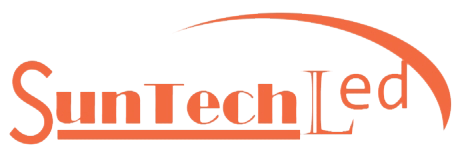# How to know whether the UV LED lamp bead is at a 30° angle, 60° angle, or full angle?

Views: 0     Author: Site Editor     Publish Time: 2020-09-18      Origin: Site

Those who know about optics know that there are generally three "angles" in optics, namely the beam angle, the shading angle, and the cut-off angle. Which "angle" does the "30° angle" of the 30° angle UVLED lamp bead refer to? Don't worry, let's take a look at the introduction of these three corners.Beam angle refers to the angle between two directions where the luminous intensity is equal to 50% of the maximum light intensity on a plane perpendicular to the center line of the beam, as shown in the figure above.

How to determine the position of the 50% light intensity point?

According to the law of cosine and the law of inverse square ratio, the illuminance value Ep of the 50% light intensity point should be:Shielding angleThe shielding angle refers to the angle between the line connecting the edge of the light source and the opening edge of the reflector and the horizontal line, as shown in the figure above.

Cut-off angleThe cut-off angle is the complementary angle of the shading angle, as shown in the figure above.

Obviously, the "30° angle" in the UVLED lamp bead with a 30° angle refers to the beam angle.

How to calculate the beam angle from the light distribution curve?

The picture below is a UVLED lamp bead with LG's model LEUVA33W70TL00 and a wavelength of 385nm. From the figure, it can be calculated that the lamp bead has an angle of 120°.Here is another one, the model is LEUVA35W70RL00, the UVLED lamp bead with a wavelength of 365nm, the lamp bead has a 60° angleIn addition to distinguishing from the light distribution curve in the specification, preliminary judgment can be made from the lamp bead lens. The higher the protrusion of the lens, the smaller the angle. As shown below:##### UVC LED Disinfection